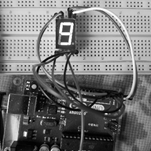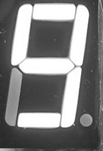• 选择你感兴趣的区域截取图片，然后下一步对你感兴趣的区域继续进行操作
• MATLAB截取图像特定区域

万次阅读 多人点赞 2019-04-14 23:22:16
MATLAB截取图像特定区域 你好！你可以仔细阅读这篇文章，了解一下MATLAB的截取图像的特定区域的方法。 二话不说先上代码 代码片. save_path='D:\picture\'; img_path_list = dir(strcat(save_path,'*....

MATLAB截取图像特定区域

你好！你可以仔细阅读这篇文章，了解一下MATLAB的截取图像的特定区域的方法。

截取程序

imcrop函数
功能：用于返回图像的一个裁剪区域。可把图像显示在一个图像窗口中。
代码片示例.

picture_1 =imcrop(picture,[x(1),y(1),abs(x(1)-x(2)),abs(y(1)-y(2))])   %切割图像，起始坐标点（x1,y1）截取到终止坐标点(x2,y2)

代码说明：picture为要裁剪的图片
picture_1裁剪后的图片
x(1),y(1)裁剪起始坐标点
abs(x(1)-x(2)),abs(y(1)-y(2))裁剪长度

读取图片

save_path='D:\picture\';     %图片地址文件夹
img_path_list = dir(strcat(save_path,'*.jpg'));    %仅读取文件夹中的.jpg文件
img_num=length(img_path_list);  %判断图片个数
for i = 1:img_num        %采用循环方式读取文件
picture_name =img_path_list(i).name;
%imshow(picture);
end

获取截取点坐标

ginput函数

功能: 允许用户以交互方式使用鼠标选定要剪切的区域以此获取两个坐标点并以矩形方式裁剪

[x,y] = ginput(n)   %n为你想选的点的个数，鼠标点的点的横坐标和纵坐标就会保存到[x,y]中。然后再输出x,y便可获取在图片上需要截取的点的坐标！

完整代码

代码片.

save_path='D:\picture\';

img_path_list = dir(strcat(save_path,'*.jpg'));

img_num=length(img_path_list);   %判断图片个数

for i = 1:img_num     %因为拍照片的时候固定好了位置所以用一个for循环就可以截取出所有的图片的数字

picture_name =img_path_list(i).name;

imshow(picture);

[x,y]=ginput(2);   %先用的ginput函数获取图片中数字的起始坐标

%x=[1.0e+03 *0.7145,1.0e+03 *1.0305];

%y=[234.5000,670.5000];

picture_1 =imcrop(picture,[x(1),y(1),abs(x(1)-x(2)),abs(y(1)-y(2))]);  %切割图像，起始坐标点（x1,y1）截取到终止坐标点(x2,y2)

imwrite(picture_1,[num2str(i),'.jpg']);%将图片保存在程序所在文件夹中

end

截取前截取后展开全文• 目前，实验矢量网络分析会测量得到数据图片，但是计算时，我们需要将其数据提取出来。本程序给出指定部位截取吗，同时将指定部位上的数字保存下来。
• MATLAB语言代码，可以将图片的某指定部分截取出来，
• pic = imread('1.jpg'); imshow(pic);...%利用imcrop函数对图像进行切割，输入参数是一个定点坐标， %从该定点出发向右abs(x(1)-x(2))，向下abs(y(1)-y(2))的区域进行切割 figure,imshow(pic_1);
imshow(pic);
[x,y] = ginput(2);    %确定图像上的两点利用ginput函数，返回值是两点的坐标
pic_1 = imcrop(pic,[x(1),y(1),abs(x(1)-x(2)),abs(y(1)-y(2))]);

%利用imcrop函数对图像进行切割，输入参数是一个定点坐标，

%从该定点出发向右abs(x(1)-x(2))，向下abs(y(1)-y(2))的区域进行切割

figure,imshow(pic_1);
imwrite(pic_1,'1.jpg');

原始图像中间过程：切割后的图像展开全文matlab 图像识别
• 设置默认工作路径 一般来说MATLAB的默认工作路径是安装目录下的bin目录,但是,把这作为自己的工作目录很不方便,以为里面已经有很多安装文件了,容易混淆: 每次打开再更改路径又太麻烦,所以最好 ...Linux tar打包命令...

MATLAB自定义配置

1.设置默认工作路径 一般来说MATLAB的默认工作路径是安装目录下的bin目录,但是,把这个作为自己的工作目录很不方便,以为里面已经有很多安装文件了,容易混淆: 每次打开再更改路径又太麻烦,所以最好 ...

Linux tar打包命令

Linux tar打包命令: 范例一:将整个 /etc 目录下的文件全部打包成为 /tmp/etc.tar [root@linux ~]# tar -cvf /tmp/etc.tar /etc &lt ...

LeetCode&lpar;1&rpar; －Two Sum

题目要求很简单,给你一个数组(例如,nums = [2,7,11,15])和一个target(target = 9),找到数组里两个数相加后能得到target的这两个数的index.在本例中,返回的应 ...

Fixing the Great Wall

题意: 在一条线上,有n个坏的地方要修机器人修,机器人的移动速度V,若坏的地方立即被修花费ci,若没修,每单位时间增加d,出去机器人的开始位置,求修完n个地方的最小花费. 分析: 非常经典,求解“未来 ...

【原创】Android 系统稳定性 - ANR(二)

文章都为原创,转载请注明出处,未经允许而盗用者追究法律责任. 很久之前写的了,留着有点浪费,共享之.编写者:李文栋P.S. OpenOffice粘贴过来后格式有些混乱. 1.2 如何分析ANR问题 引 ...

【安装】python3&period;4版安装与2&period;x共存问题

Linux-yum只下载不安装

通过yum命令只下载rpm包不安装 经常遇到服务器没有网络的情况下部署环境,或者创建自己的 yum 仓库等.每次都是在网上搜搜搜,都是五花八门,自己整理了下自己用到的以下三种方式,这里没有太多废话,只 ...

2、ansilbe常用模块详解及inventory介绍

Ansible ansible格式: ansible [-f forks] [-m module_name] [-a args] args: 用法 key=v ...

【Excel技能】字符串包含某字符串个数？替换许多组字符串？

=len(单元格A)-len(substitute(单元格A,某字符串,)) 原理:将某字符串替换成空,前后字符串长即为减去的这个字符串长度,这个字符串出现个数=前后字符串长度之差/这个字符串长度 = ...

Eclipse------使用Maven install出错：编码GBK的不可映射字符

使用Maven install时报错:编码GBK的不可映射字符 原因:Maven默认使用GBK进行编码 解决方法: 在pom.xml文件中添加如下代码即可

展开全文• 就可以轻松提取到图像中100*300大小矩形区域图像那么有时候需要提取图像中圆形区域图像，其实应用圆的表达式就可以很容易求取下面是自己用matlab写的一个提取圆形区域图像的函数，可供大家参考：functionIMG_Out...

图像中提取圆形区域子图像

在图像中提取矩形区域非常容易，应用冒号表达式即可：

例如：

IMG_Out=IMG_In(300:400,400:700);

就可以轻松提取到图像中

100*300

大小矩形区域的图像

那么有时候需要提取图像中圆形区域的图像，其实应用圆的表达式就可以很容易求取

下面是自己用

matlab

写的一个提取圆形区域图像的函数，可供大家参考：

function

%IMG_In

是输入图像，可以是灰度图，也可以使

RGB

格式的彩色图

要截取圆形区域的半径值

%Center

圆心的坐标，

Center

(

1

)为

x

坐标，

Center

(

2

)为

y

坐标，

Center

1x2

结构

%IMG_Out

输出图像

%2015.6.11

郑州大学光电信息科学研究所

if

[m,n,~]=size(IMG_In);

[X,Y]=meshgrid(1:n,1:m);

R_temp=sqrt((X-Center(1)).^2+(Y-Center(2)).^2);

if

(numel(size(IMG_In))>2)

R_Out=R_temp1.*im2double(IMG_In(:,:,1));

G_Out=R_temp1.*im2double(IMG_In(:,:,2));

B_Out=R_temp1.*im2double(IMG_In(:,:,3));

IMG_Out(:,:,1)=R_Out;

IMG_Out(:,:,2)=G_Out;

IMG_Out(:,:,3)=B_Out;

else

IMG_Out=R_temp1.*im2double(IMG_In);

end

else

disp(

);

end

imshow(IMG_Out);

展开全文• 利用MATLAB截取图片某个区域

万次阅读 多人点赞 2015-06-30 15:01:20
pic = imread('1.jpg'); imshow(pic); [x,y] = ginput(2); %确定图像上的两点利用ginput函数，返回值是两点的坐标 ...%利用imcrop函数对图像进行切割，输入参数是一个定点坐标， %从该定点出发向右amatlab 图像处理
• Matlab里面根据鼠标的响应，截取rect区域图像，并且保存图像。代码非常简单，里面没有做越界判断。clc;clear;% label and rectForder = [pwd '\images\'];files = dir([Forder,'*.png']);L = length(files);num = 0;...
• 图像中心定点 进行区域分割，时候用于人脸图像的局部分割图像处理
• % 提取图像文件后缀 if strcmp(suffix,'pgm') | strcmp(suffix,'raw') % 要求是pgm格式或者raw格式 disp(sprintf('\nopens %s file\n',filename)); fp = fopen(filename,'rb','b'); % "Big-endian" byte order. if...matlab 图像处理 图片
• %第步：从图片选取矩形框区域 I = imread('o.png'); [A,rect] = imcrop(I); imshow(A); rect %第二步：根据rect确定：在原图绘制的矩形的坐标，注意rect的格式[m n l k]->[(m,n) (m+l,n+k)]->[(n,m) (n+k...
• Matlab从图像中选取矩形区域

万次阅读 热门讨论 2016-03-18 16:37:01matlab
• matlab自带了一些用于图像交互式操作的函数，在这里记录一下； getpt 作用：你鼠标点击...p返回了四个数，依次为左上角像素的列，左上角像素的行，列长，行长，由这个四个值就能确定一个矩形区域。 示例： img =matlab图片
• 用matlab实现图像截取功能的小程序，简单简洁，在Matlab上运行m文件即可，有友好的用户界面，手动框定图像图像区域点击保存即可实现截取功能
• matlab图像剪裁命令imcrop（）

千次阅读 2021-01-17 17:20:51
其中，I、X、RGB分别对应灰度图像、索引图像、RGB图像的数据矩阵，MAP为索引图像颜色表，I2、X2、RGB2分别为各自输入矩阵所对应的输出矩阵。而RECT为可选参数，格式为[XMIN YMIN WIGTH HEIGHT].例如[20 20 40 40]，...
• matlab程序批量根据鼠标点两下选中图片矩形区域剪切保存 可直接运行
• 可以用于在matlab中根据曲线画出阴影，用于表示置信区间的种方法。
• MATLAB图像处理工具箱(Image ProcessingToolbox)的GUI函数，有一些用来在图像或坐标轴上选择矩形或椭圆区域的函数，使用示例如下：figure,imshow('cameraman.tif');h=imrect(gca,);api=iptgetapi(h)...
• Matlab | 提取图像ROI任意形状

千次阅读 2020-12-19 08:15:14
前言本文给出在matlab中如何提取图像任意形状的感兴趣(ROI)区域的程序及分析。程序程序如下：clc;clear;I=imread('1.bmp'); % 读取一张图片imshow(I); % 显示原始图像i=rgb2gray(I); % 变为灰度图像[c,r]=ginput(20)......

用matlab截取图像中一个区域matlab 订阅